# Busemann function

(diff) ← Older revision | Latest revision (diff) | Newer revision → (diff)

A concept of function which measures the distance to a point at infinity. Letbe a Riemannian manifold. The Riemannian metric induces a distance function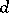on. Letbe a ray in, i.e., a unit-speed geodesic line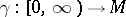such that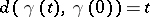for all. The Busemann function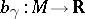with respect tois defined by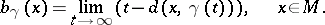Since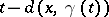is bounded above by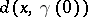and is monotone non-decreasing in, the limit always exits. It follows that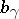is a Lipschitz function with Lipschitz constant. The level surfaces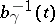of a Busemann function are called horospheres. Busemann functions can also be defined on intrinsic (or length) metric spaces, in the same manner. Actually, H. Busemann [a2] first introduced them on so-called-spaces and used them to state the parallel axiom on straight-spaces (cf. also Closed geodesic).

Ifhas non-negative sectional curvature,is convex, see [a3]. Ifhas non-negative Ricci curvature,is a subharmonic function, see [a4]. Ifis a Kähler manifold with non-negative holomorphic bisectional curvature,is a plurisubharmonic function, see [a7]. Ifis a Hadamard manifold,is a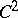concave function, see [a9], [a2], and, moreover, the horospheres are-hypersurfaces, see [a9]. On the Poincaré model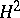of the hyperbolic space, the horospheres coincide with the Euclidean spheres inwhich are tangent to the sphere at infinity. On Hadamard manifolds, it is more customary to call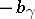the Busemann function instead of.

More recently, M. Gromov [a1] introduced a generalization of the concept of Busemann function called the horofunction. Let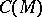be the set of continuous functions onand let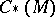the quotient space ofmodulo the constant functions. Use the topology oninduced from the uniform convergence on compact sets and its quotient topology on. The embedding ofintodefined by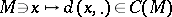induces an embedding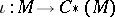. The closure of the image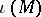is a compactification of(cf. also Compactification). According to [a1], [a8], a horofunction is defined to be a class (or an element of a class) in the topological boundaryofin. Any Busemann function is a horofunction. For Hadamard manifolds, any horofunction can be represented as some Busemann function, see [a1]. However, this is not necessarily true for non-Hadamard manifolds. Horofunctions have been defined not only for Riemannian manifolds but also for complete locally compact metric spaces.

Letbe a complete non-compact Riemannian manifold with non-negative sectional curvature, and for, let,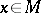, whereruns over all rays emanating from. Then,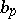is a convex exhaustion function, see [a3], that is, a functiononsuch that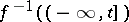is compact for any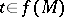. The functionplays an important role in the first step of the Cheeger–Gromoll structure theory for, see [a3]. Any Kähler manifold admitting a strictly plurisubharmonic exhaustion function is a Stein manifold, see [a5]. This, together with the use of Busemann functions or, yields various sufficient conditions for a Kähler manifold to be Stein; see, for example, [a7], [a17]. Some results for the exhaustion property of Busemann functions are known, see [a14], [a15], [a12], [a13]. For a generalization of the notion of a horofunction and of, see [a18]. A general reference for Busemann function and its related topics is [a16].

How to Cite This Entry:
Busemann function. Encyclopedia of Mathematics. URL: http://encyclopediaofmath.org/index.php?title=Busemann_function&oldid=13719
This article was adapted from an original article by T. Shioya (originator), which appeared in Encyclopedia of Mathematics - ISBN 1402006098. See original article